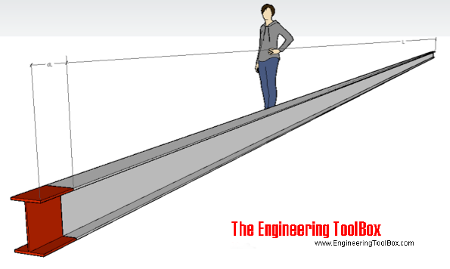Engineering ToolBox - Resources, Tools and Basic Information for Engineering and Design of Technical Applications!

# Linear Thermal Expansion

## Linear temperature expansion - online calculator

When an object is heated or cooled, its length changes by an amount proportional to the original length and the change in temperature. The linear thermal expansion - the change in length - of an object can be expressed as

dl = L0 α (t1 - t0)                                (1)

where

dl = change in object length (m, inches)

L0 = initial length of object (m, inches)

α =  linear expansion coefficient (m/moC, in/inoF)

t0 = initial temperature (oC, oF)

t1 = final temperature (oC, oF)

The final length of the object can be calculated as

L1 = L0 + dl

= L0 + L0 α (t1 - t0)                                (2)

where

L1 = final length of object (m, inches)

Note! - linear expansion coefficients for most materials varies with temperature.

For wider temperature ranges - calculate for smaller spans and integrate the results.

### Online Thermal Linear Expansion Calculator

L0 - initial length of object (m, inches)

α - linear expansion coefficient (m/moC, in/inoF)

t0 - initial temperature (oC, oF)

t1 - final temperature (oC, oF)

#### Linear Temperature Coefficients - α - for some common metals

• aluminum : 0.000023 (m/moC) (23 μm/moC)
• steel: 0.000012 (m/moC) (12 μm/moC)
• copper: 0.000017 (m/moC) (17 μm/moC)
• more coefficients ..
• more metals ..

### Example - Expansion of an Aluminum BeamAn aluminum construction is designed for temperatures ranging -30oC to 50oC. If a beam's length is 6 m when assembled at 20oC - the shortest final length of the beam at minimum temperature -30oC can be calculated as

L1 = (6 m) + (6 m) (0.000023 m/moC) ((-30 oC) - (20 oC))

=  5.993 m

The longest final length of the beam at maximum temperature 50oC can be calculated as

L1 = (6 m) + (6 m) (0.000023 m/moC) ((50 oC) - (20 oC))

=  6.004 m

### Superficial Expansion

The amount by which a unit area of a material increases when the temperature is raised by one degree is called the coefficient of superficial (area) expansion.

### Cubic Expansion

The amount by which a unit volume of a material increases when the temperature is raised by one degree is called the coefficient of cubic expansion.

## Engineering ToolBox - SketchUp Extension - Online 3D modeling!

Add standard and customized parametric components - like flange beams, lumbers, piping, stairs and more - to your Sketchup model with the Engineering ToolBox - SketchUp Extension - enabled for use with the amazing, fun and free SketchUp Make and SketchUp Pro .Add the Engineering ToolBox extension to your SketchUp from the SketchUp Pro Sketchup Extension Warehouse!

Translate

## Privacy

We don't collect information from our users. Only emails and answers are saved in our archive. Cookies are only used in the browser to improve user experience.

Some of our calculators and applications let you save application data to your local computer. These applications will - due to browser restrictions - send data between your browser and our server. We don't save this data.

## Citation

• Engineering ToolBox, (2008). Linear Thermal Expansion. [online] Available at: https://www.engineeringtoolbox.com/linear-thermal-expansion-d_1379.html [Accessed Day Mo. Year].

Modify access date.

. .

#### Scientific Online Calculator10 15